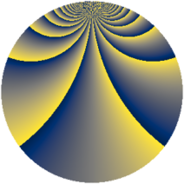# Properties

 Label 1805.2.kLevel $1805$ Weight $2$ Character orbit 1805.k Rep. character $\chi_{1805}(606,\cdot)$ Character field $\Q(\zeta_{9})$ Dimension $684$ Sturm bound $380$

# Related objects

## Defining parameters

 Level: $$N$$ $$=$$ $$1805 = 5 \cdot 19^{2}$$ Weight: $$k$$ $$=$$ $$2$$ Character orbit: $$[\chi]$$ $$=$$ 1805.k (of order $$9$$ and degree $$6$$) Character conductor: $$\operatorname{cond}(\chi)$$ $$=$$ $$19$$ Character field: $$\Q(\zeta_{9})$$ Sturm bound: $$380$$

## Dimensions

The following table gives the dimensions of various subspaces of $$M_{2}(1805, [\chi])$$.

Total New Old
Modular forms 1260 684 576
Cusp forms 1020 684 336
Eisenstein series 240 0 240

## Trace form

 $$684 q + 6 q^{3} + 6 q^{4} + 12 q^{6} + 18 q^{8} + 18 q^{9} + O(q^{10})$$ $$684 q + 6 q^{3} + 6 q^{4} + 12 q^{6} + 18 q^{8} + 18 q^{9} + 6 q^{10} + 12 q^{12} + 6 q^{13} - 12 q^{14} - 18 q^{16} - 36 q^{18} + 18 q^{21} - 24 q^{22} - 12 q^{23} - 6 q^{24} + 42 q^{26} + 18 q^{27} + 36 q^{28} - 6 q^{29} + 48 q^{30} - 12 q^{31} - 60 q^{32} + 36 q^{33} - 12 q^{34} + 6 q^{35} - 54 q^{36} + 24 q^{37} - 120 q^{39} + 12 q^{40} + 36 q^{41} - 30 q^{42} + 42 q^{43} - 12 q^{44} - 30 q^{46} - 54 q^{47} + 30 q^{48} - 366 q^{49} + 6 q^{50} + 18 q^{51} + 30 q^{52} - 12 q^{53} + 36 q^{54} + 72 q^{56} - 96 q^{58} - 36 q^{59} - 12 q^{60} + 24 q^{61} + 12 q^{62} - 78 q^{63} - 336 q^{64} + 6 q^{65} - 6 q^{66} + 24 q^{67} - 12 q^{68} - 42 q^{69} - 72 q^{70} - 12 q^{71} + 48 q^{72} - 30 q^{73} + 54 q^{74} - 12 q^{75} + 36 q^{77} - 24 q^{78} + 42 q^{79} - 48 q^{80} - 42 q^{81} + 72 q^{82} + 24 q^{83} - 36 q^{84} - 48 q^{85} - 30 q^{86} + 120 q^{87} - 12 q^{88} + 60 q^{89} + 30 q^{90} - 24 q^{91} + 108 q^{92} + 36 q^{94} - 60 q^{96} + 60 q^{97} - 36 q^{98} + 90 q^{99} + O(q^{100})$$

## Decomposition of $$S_{2}^{\mathrm{new}}(1805, [\chi])$$ into newform subspaces

The newforms in this space have not yet been added to the LMFDB.

## Decomposition of $$S_{2}^{\mathrm{old}}(1805, [\chi])$$ into lower level spaces

$$S_{2}^{\mathrm{old}}(1805, [\chi]) \cong$$ $$S_{2}^{\mathrm{new}}(19, [\chi])$$$$^{\oplus 4}$$$$\oplus$$$$S_{2}^{\mathrm{new}}(95, [\chi])$$$$^{\oplus 2}$$$$\oplus$$$$S_{2}^{\mathrm{new}}(361, [\chi])$$$$^{\oplus 2}$$